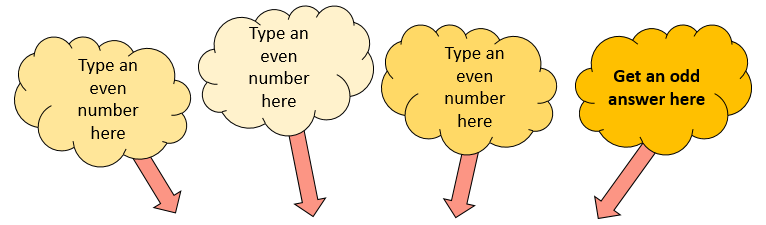# Mix and Math

##### Level 1Level 2Level 3ChallengeDescriptionMore Number÷×=This challenge involves finding an even number that when divided by a different even number then multiplied by another different even number produces an odd answer. Type your guesses into the boxes above then click the check button. Ticks on the left of the equals sign indicate your numbers are even. A tick on the right indicates that the number on the right is odd and is the result of the calculation on the left. Get four ticks to earn a trophy.

Check

Well done.

You have found four numbers that work. Are there any other solutions?

## Description

Determine the nature of adding, subtracting and multiplying numbers with specific properties.

CloseLevel 1 - Even and Odd Numbers

Level 2 - Positive and Negative Numbers

Level 3 - Prime and Composite Numbers

Challenge - Can you find numbers that will satisfy the Mix And Math Challenge?

Answers to this exercise are available lower down this page when you are logged in to your Transum account. If you don’t yet have a Transum subscription one can be very quickly set up if you are a teacher, tutor or parent.

## Definitions

Even numbers are exactly divisible by two.

Odd numbers are not exactly divisible by two.

Positive numbers are greater than zero.

Negative numbers are less than zero.

Prime numbers only have two factors.

Composite numbers have more than two factors.

The solutions to this and other Transum puzzles, exercises and activities are available here when you are signed in to your Transum subscription account. If you do not yet have an account and you are a teacher, tutor or parent you can apply for one by completing the form on the Sign Up page.